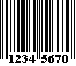## EAN-8 Specification

This symbology is a shortened version of EAN-13. EAN-8 encodes 8 numeric digits consisting of two country code digits, five data digits and one check digit.

### Structure

Each EAN bar code character is comprised of two bars and two spaces. The wide elements are integer multiples of the narrow elements (X-dimension), which means that one, two, three, or four narrow elements together create one wide element. There are a total of seven elements in each EAN character.### Symbol Creation

A complete EAN 8 symbol includes the start character, the left side data field, the center bar character, the right side data field, the check digit, the stop character, and the area immediately surrounding the symbol (quiet zones). The start character and stop character may also be referred to as the left guard bar and the right guard bar.

### Start Character

The start character (left guard bar) is used at the beginning of the symbol to provide the bar code reader with start instructions.

### Left Side Data Field

The left side data field consists of four odd parity characters. Odd parity characters are comprised of three or five bars.

### Right Side Data Field

The right side data field consists of four even parity characters: three digits plus the modulus 10 check digit.

### Check Digit Calculation

The check digit is the last character in the right side data field, and is used to verify that the left and right side data fields have been coded properly.

```Step 1: Designate the least significant digit postion as odd.

7 9 0 1 2 5 8
O E O E O E O

Step 2: Sum all the digits in the odd (O) positions

7+0+2+8 = 17

Step 3: Multiply the result by 3

17 * 3 = 51

Step 4: Sum all the digits in the even (E) positions.

9+1+5 = 15

Step 5: Add the result of Step 2 with the result of Step 4.

51 + 15 = 66

Step 6: Subtract from the next higher multiple of 10.

70 - 66 = 4

In this example, the check digit is 4.
```